##### 几何工具: 分级测试

1:00:00

1.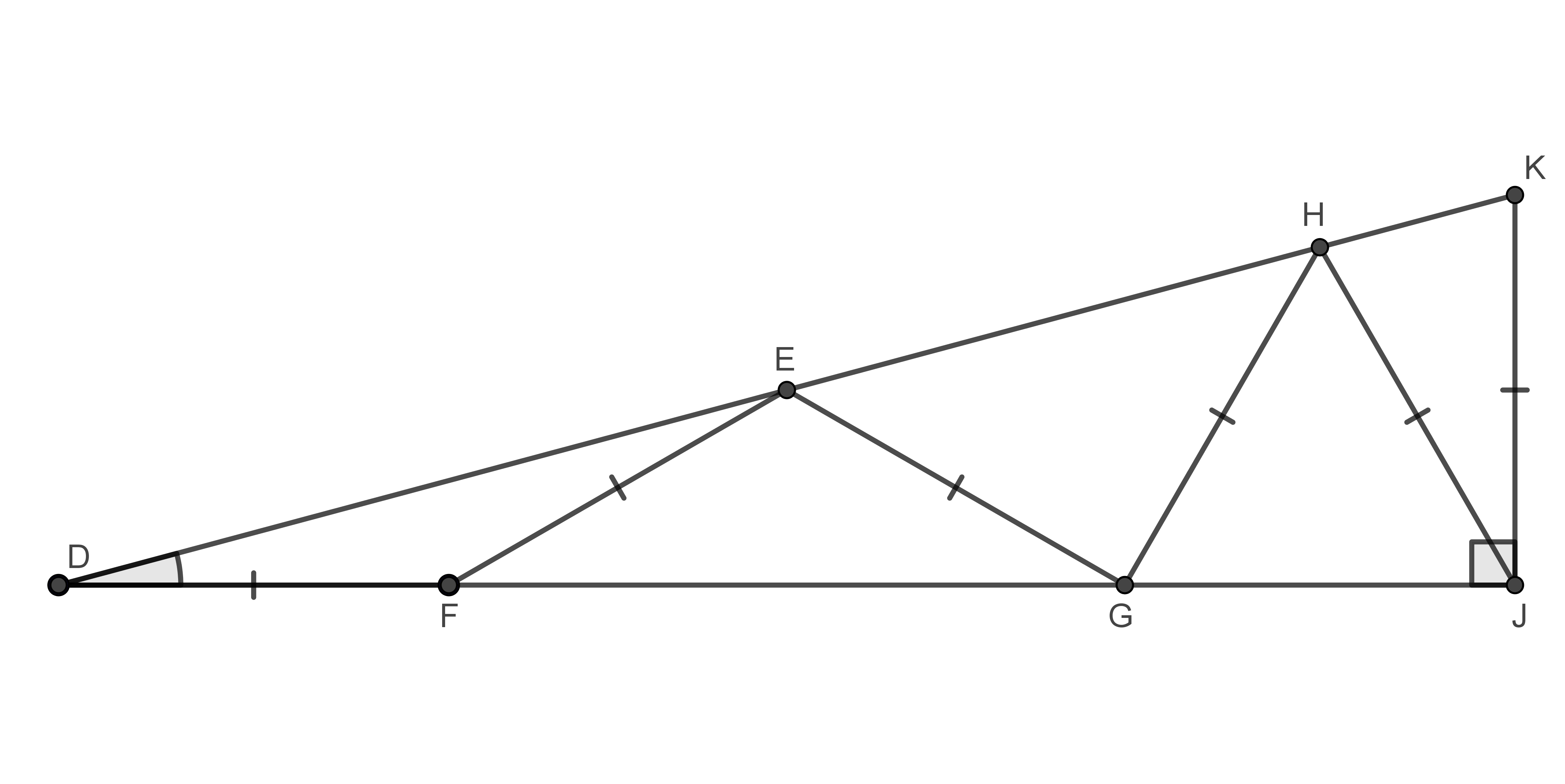What is the measure of $$\angle D?$$ Express your answer in degrees rounded to the nearest whole degree.

If your answer is $$31.4^{\circ},$$ you would enter: $$31$$

2.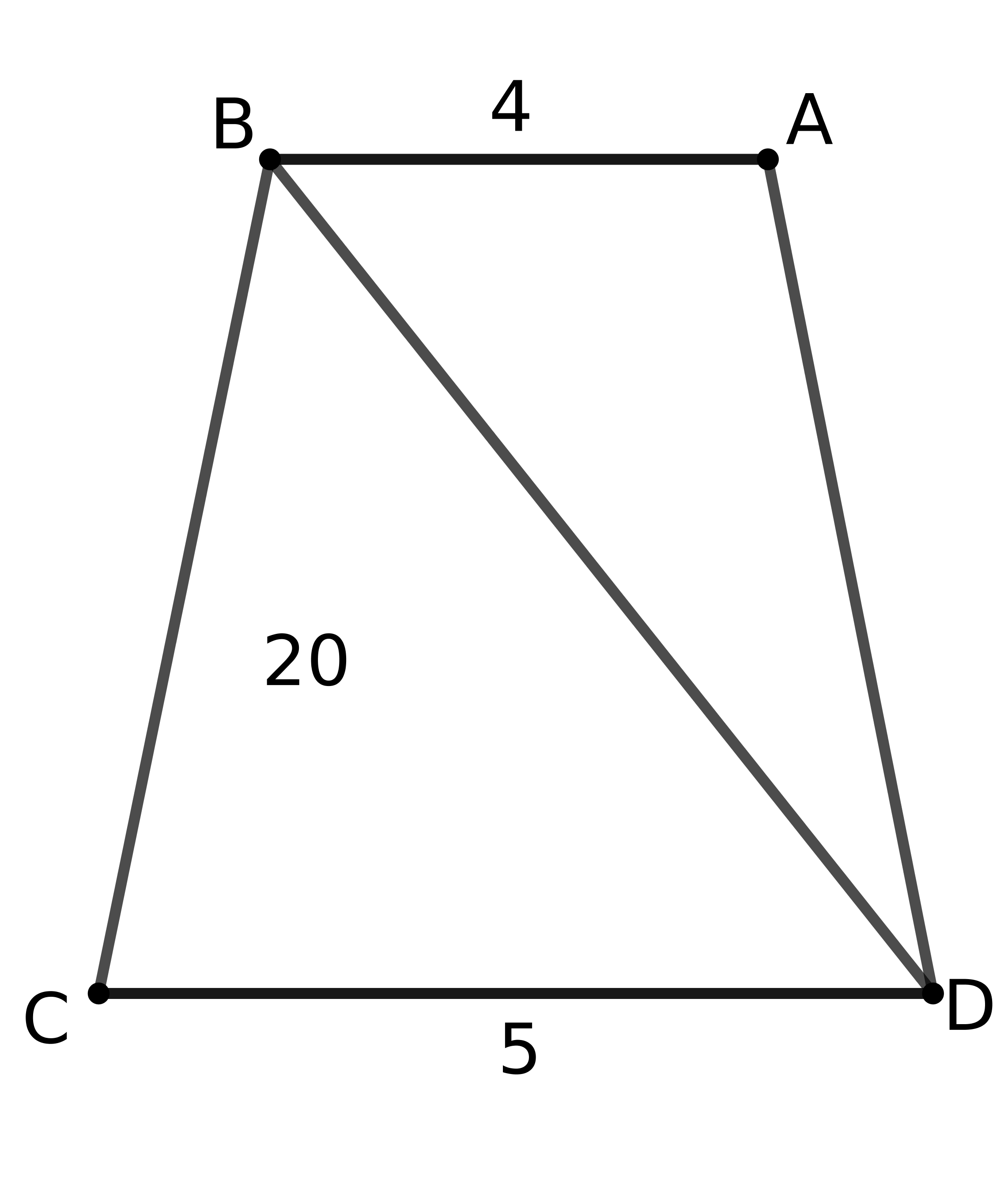Sides $$AB$$ and $$CD$$ are parallel. The area of $$\bigtriangleup BCD$$ is $$20.$$ What is the area of $$\bigtriangleup ABD?$$ (The diagram is not drawn to scale.)

3.

Suppose $$B$$ and $$C$$ are endpoints of a diameter of a circle. If $$A$$ is another point on this circle, and $$AB = 12$$ and $$AC = 16,$$ what is the radius of the circle?

4.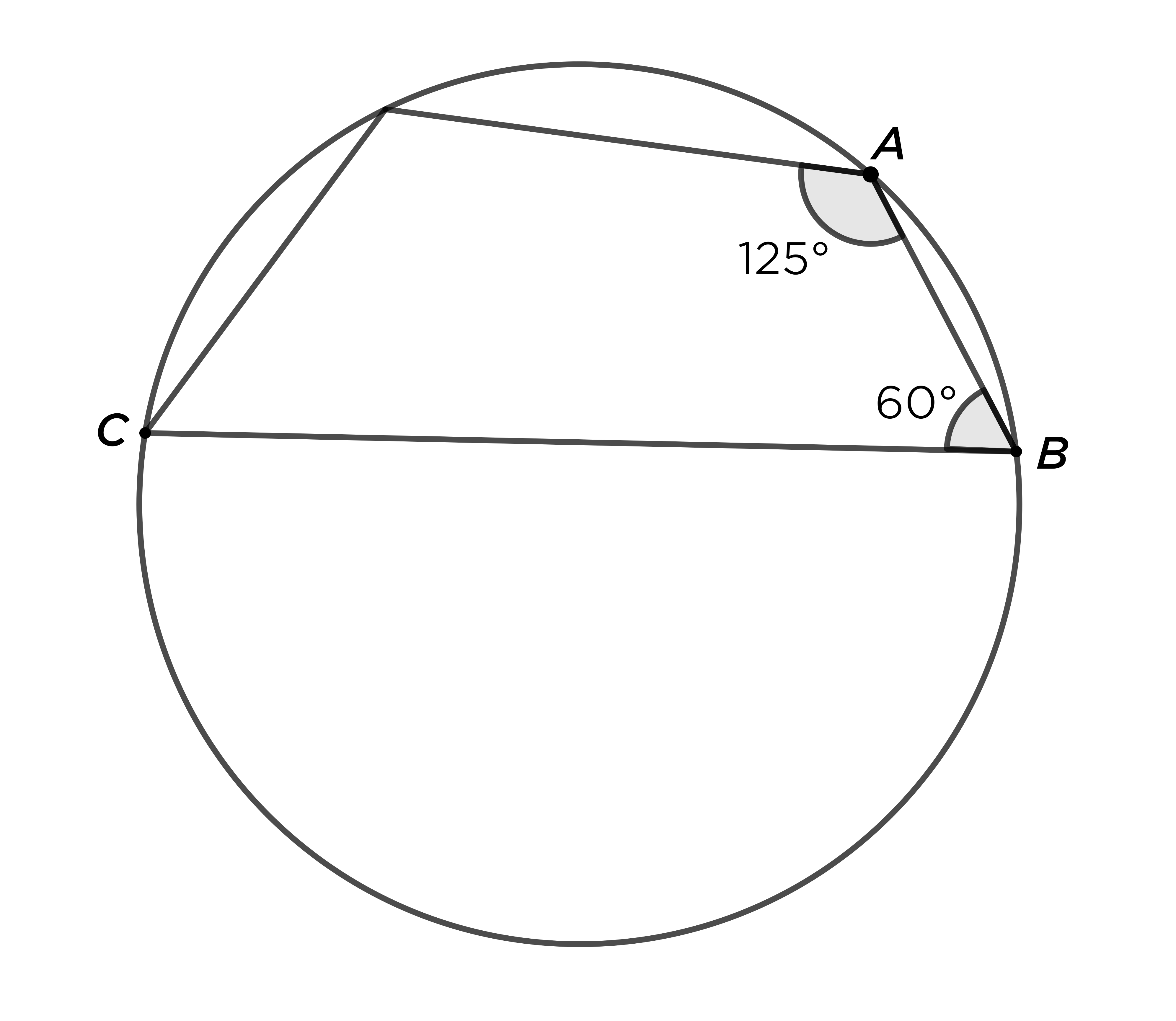If the four corners of this quadrilateral are all on the circle, and $$\angle A = 125^\circ$$ and $$\angle B = 60^\circ,$$ how many degrees are in $$\angle C?$$

5.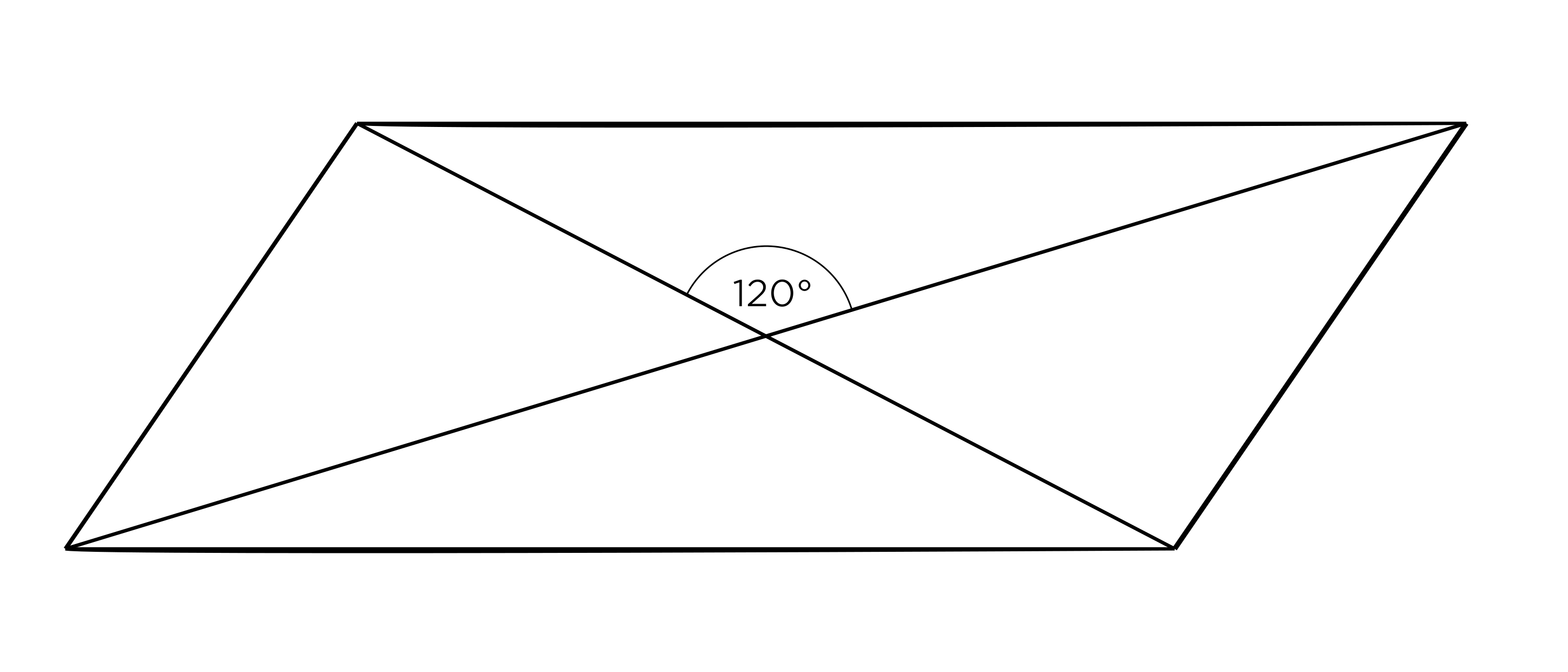Let $$a$$ be the area of this parallelogram with diagonals $$8$$ and $$4.$$ What is $$a^2?$$

6.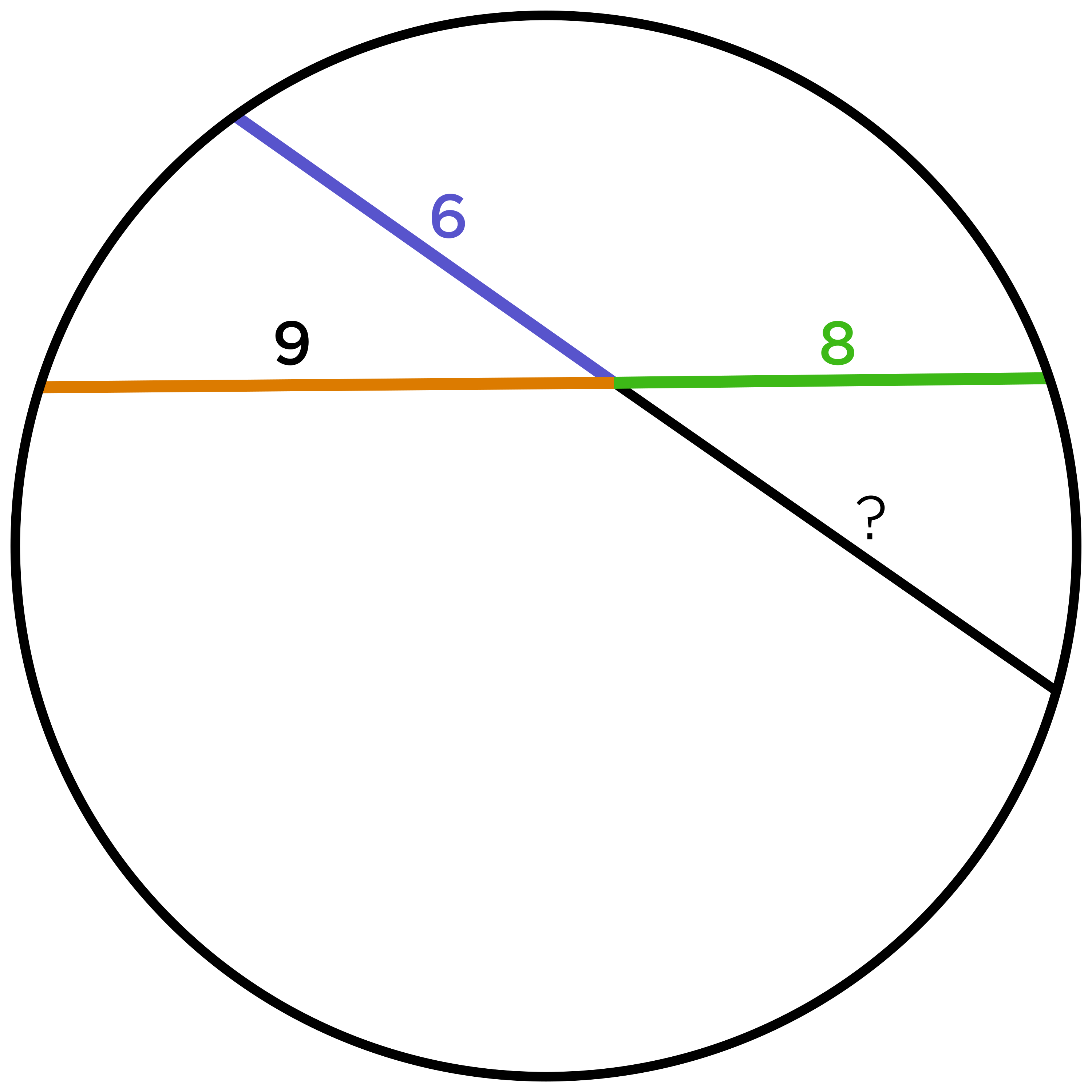In a circle with radius $$12$$, two chords intersect, creating segments of length $$6,$$ $$8,$$ and $$9,$$ as shown. What is the length of the missing segment?

7.

How many different triangles with integer side lengths have a perimeter of $$8?$$

8.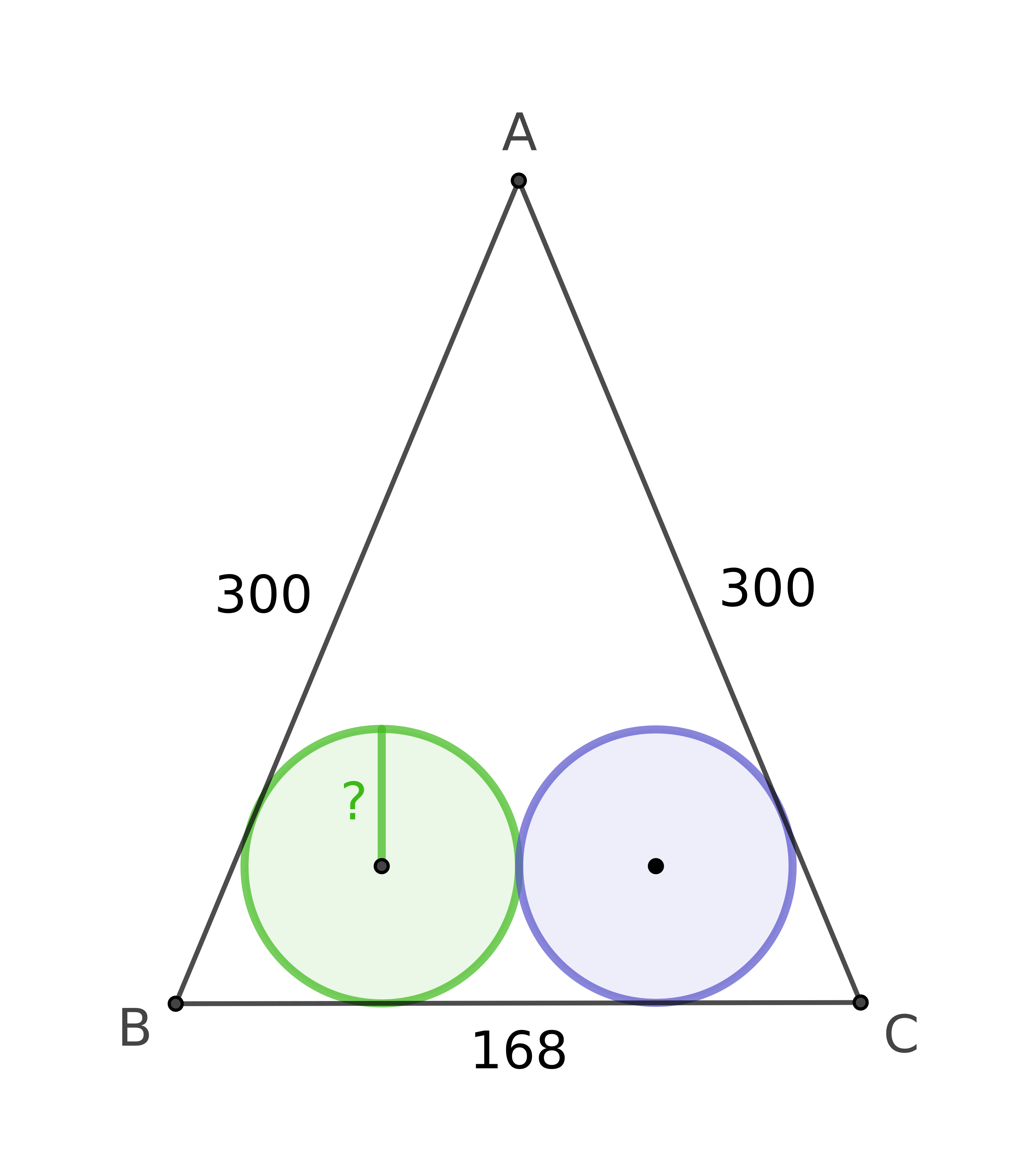An isosceles triangle has sides of $$AB = 300,$$ $$AC = 300,$$ and $$BC = 168.$$ There are two inscribed circles with the same radius, both internally tangent to the triangle along $$\overline{BC}.$$ What is the length of the radius of the circles?

9.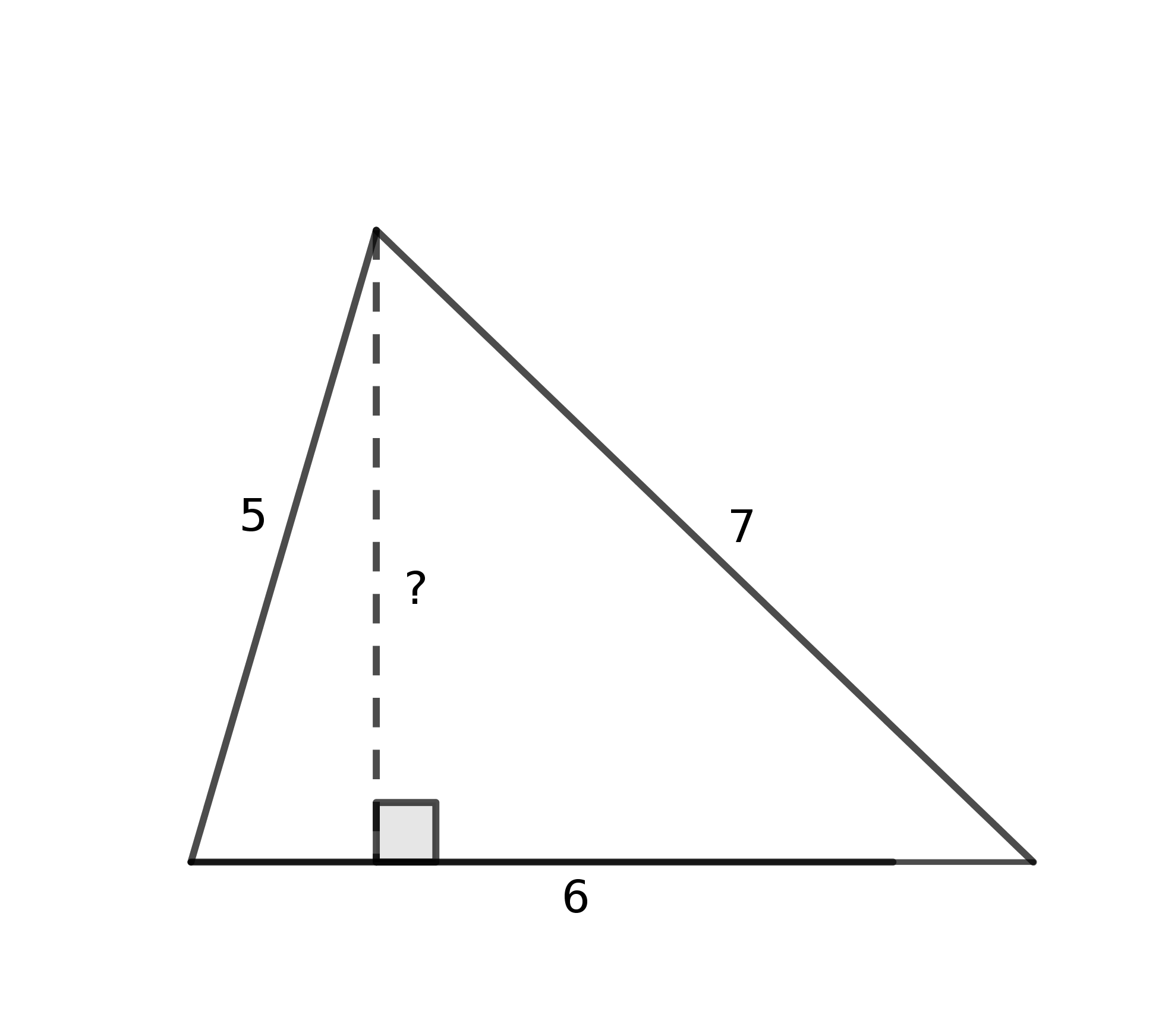What is the square of the height of this triangle?

10.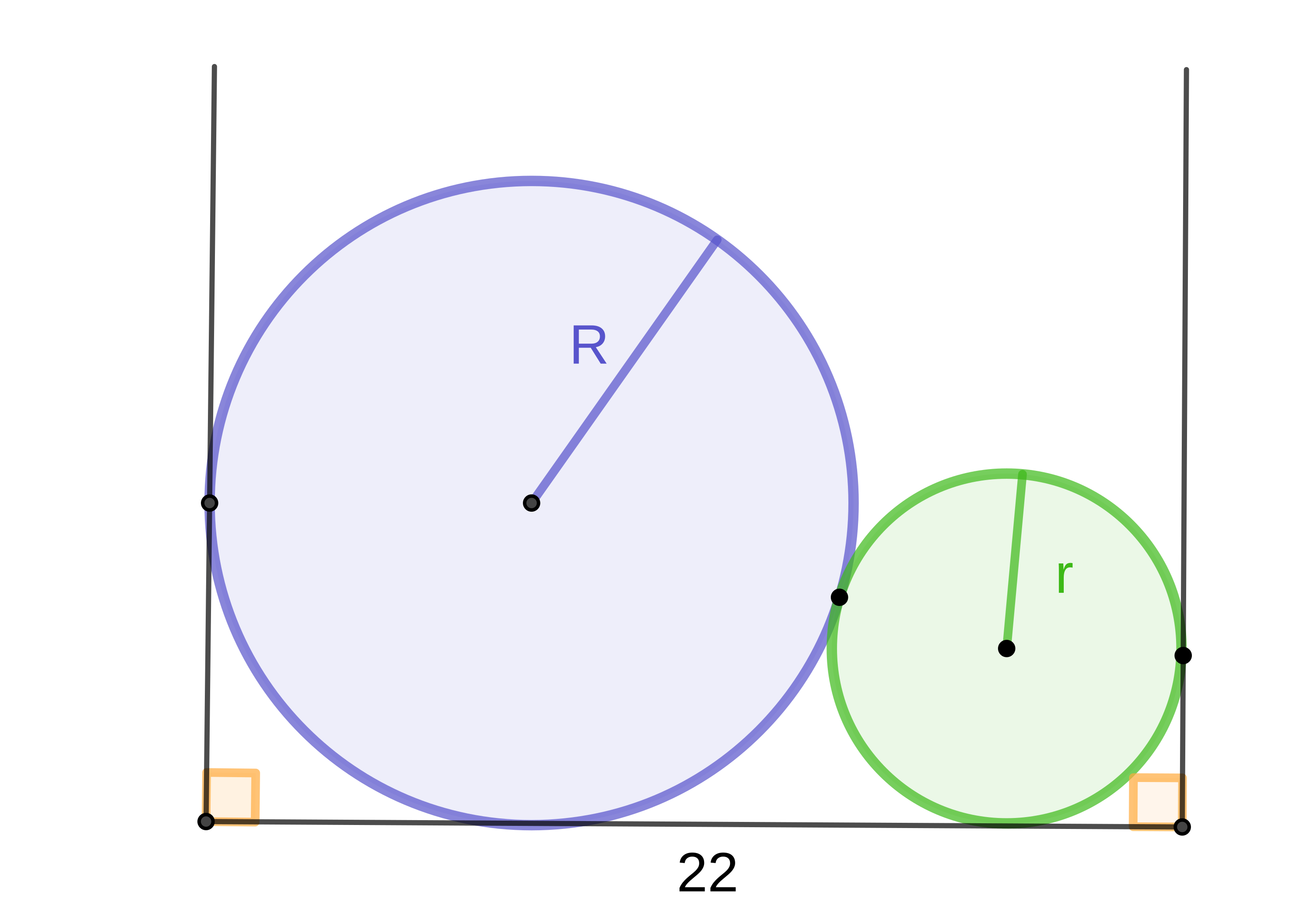Two balls fit snugly between two walls that are $$22$$ inches apart. The sum of the radii of the balls is $$12.$$ What is the product of the two radii?